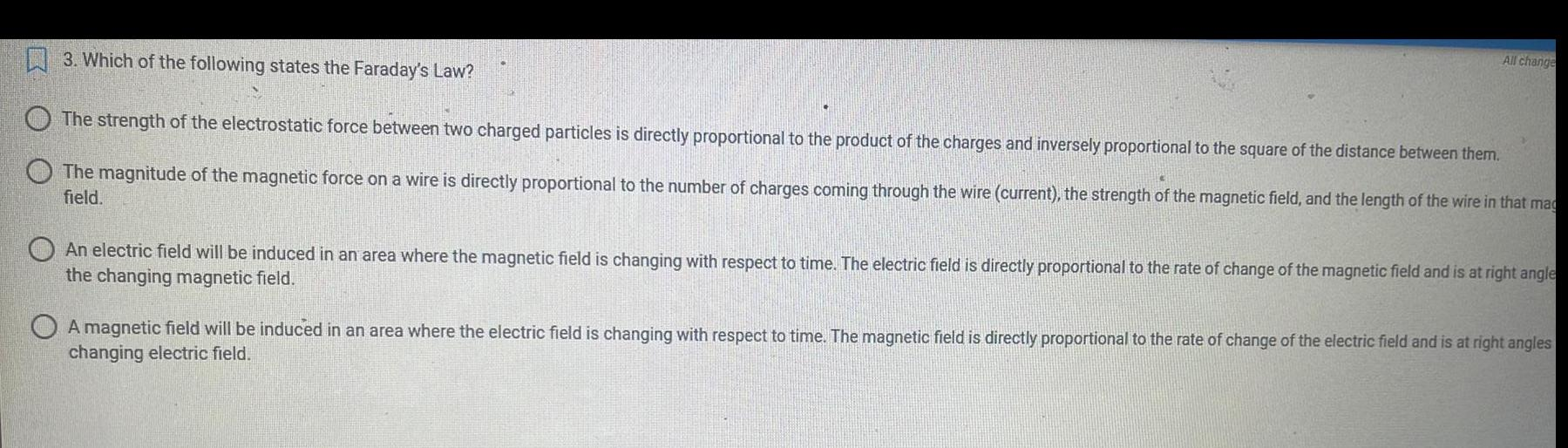Question:

# 3. Which of the following states the Faraday's Law? The

Last updated: 8/7/20223. Which of the following states the Faraday's Law? The strength of the electrostatic force between two charged particles is directly proportional to the product of the charges and inversely proportional to the square of the distance between them. The magnitude of the magnetic force on a wire is directly proportional to the number of charges coming through the wire (current), the strength of the magnetic field, and the length of the wire in that mag field. All change An electric field will be induced in an area where the magnetic field is changing with respect to time. The electric field is directly proportional to the rate of change of the magnetic field and is at right angle the changing magnetic field. A magnetic field will be induced in an area where the electric field is changing with respect to time. The magnetic field is directly proportional to the rate of change of the electric field and is at right angles changing electric field.